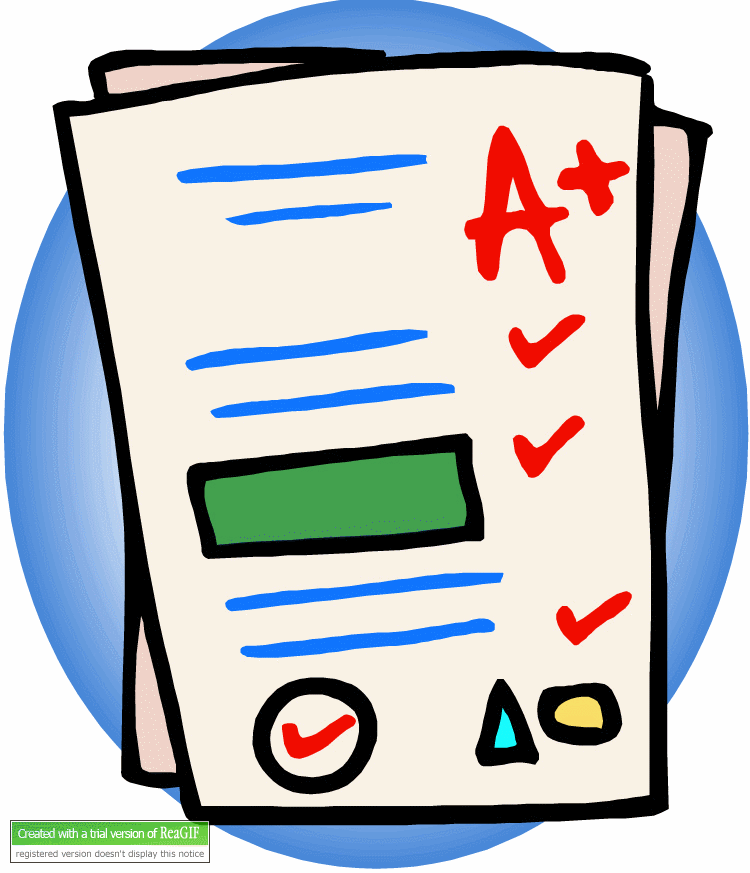# Shapes Of Polygon Quiz Questions

7 QuestionsSettingsRelated Topics
• 1.
Which quadrilateral must have congruent diagonals?
• A.

Kite

• B.

Rectangle

• C.

Parallelogram

• D.

Rhombus

• 2.
Figure ABCD is a rhombus.  The length of  is (x + 5) units, and the length of  is (2x - 3) units.Which statement best explains why the equation x + 5 = 2x - 3 can be used to solve for x?
• A.

Opposite sides of a rhombus are parallel.

• B.

Diagonals of a rhombus bisect each other.

• C.

Diagonals of a rhombus are perpendicular.

• D.

All four sides of a rhombus are congruent.

• 3.
In the figure below,  is parallel to .Which of the following statements about the figure must be true?
• A.
• B.
• C.
• D.
• 4.
Find the value of x in the following polygon
• 5.
Some cells reproduce every 40 minutes at the rate shown in the picture below.How many cells would there be at 160 minutes?
• 6.
The two squares below intersect at point C.What is the sum of the measures of  and , in degrees?
• 7.
Roderick works for JB Spas.  He is installing a circular spa that will be enclosed by a square deck.  The figure below illustrates the spa and the deck as seen from above.The radius of the spa is 3.25 feet.  What will be the perimeter, in feet, of the deck?